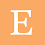## Wednesday, January 7, 2015

The process in which the heat energy of a system remains constant is called idiomatic process.

In this process there is no change in heat energy. By applying this point in the first law thermodynamics we can prove that the work done is equal to change in internal energy with an opposite sign.

For the heat energy to remain constant, the system shall be perfectly insulated from the surroundings.

As we need not keep the temperature constant we need not perform this process in slow way.

As the temperature is not constant Boyle’s law is not valid in this process.

In the following diagram we have derived the equation for the work done in adiabatic process.While deriving this equation the pressure is expressed in terms of the volume as the integration is done in terms of the volume.

Relation between pressure and temperature in a adiabatic process

The process in which heat energy of a system always remains constant is called adiabatic process.

In the PV relation we can eliminate the volume in terms of the temperature so that we can get the required relation.

Relation between volume and temperature in a adiabatic process

This relation is also derived basing on the same conditions. Here pressure has to be eliminated in terms of the temperature so that we can get the relation between the volume and temperature.

Problem and solution

If the ratio of the densities is given in a adiabatic process for a diatomic gas, find the ratio of pressures?

While solving this problem we how to convert the mass into the terms of density. We know that density is defined as the ratio of mass to its volume and that relation is used.
Further it is simply applied in between P and V relation of adiabatic process as shown below.

Problem and solution

An ideal gas is at a pressure of 1 atm pressure and the temperature of 27°C. It is compressed adiabatic early less pressure becomes eight times of its initial pressure. What is the final temperature of the system assuming that ratio specific heat is 3/2?

Problem and solution

Two moles of a Mono atomic gas at 27°C acupressure certain volume. If the volume of the gases doubled what is the work done in the adiabatic process?

We have to use the relation between the temperature and volume of a gas in adiabatic process to solve this problem as shown below.

Problem and solution

In a adiabatic process the pressure of a gas is proportional to tube of absolute temperature what is the ratio specific heats of this gas?

The above page is having another problem also. In the problem in the prophecies adiabatic heat energy is constant, temperature is variable. In a isobaric process the pressure of a system is constant.

Related Posts

#### 1 comment:

1.